Home > A2C > Chapter 11 > Lesson 11.2.2 > Problem11-61

11-61.
1. Factor and simplify each expression below. Homework Help ✎

1.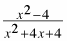2.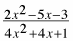3. Justify each step in simplifying the expression in part (a).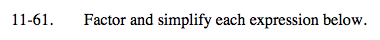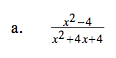$\frac{(x + 2)(x - 2)}{(x + 2)(x + 2)}$

$\frac{x - 2}{x + 2}$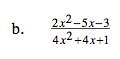See part (a).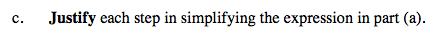Use the Distributive Property to factor and the multiplicative property of 1 to reduce.